# 1. Discretized Non-negative Matrix Factorization (dNMF)

Laboratory for Bioinformatics Research, RIKEN Center for Biosystems Dynamics Research

# Introduction

In this vignette, we consider approximating a binary or non-negative matrix as a product of two non-negative low-rank matrices (a.k.a., factor matrices).

Test data is available from toyModel.

library("dcTensor")
## Warning: no DISPLAY variable so Tk is not available
X <- dcTensor::toyModel("dNMF")

You will see that there are five blocks in the data matrix as follows.

suppressMessages(library("fields"))
image.plot(X, main="Original Data", legend.mar=8)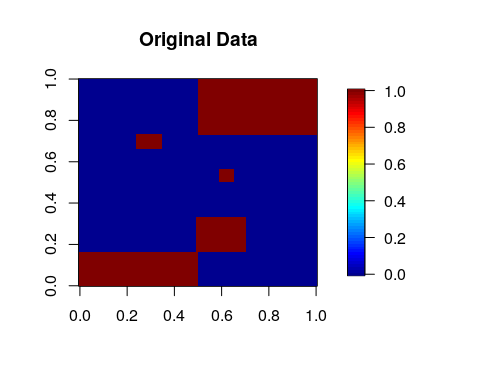# Binary Matrix Factorization (BMF)

Here, we consider the approximation of a binary data matrix $$X$$ ($$N \times M$$) as a matrix product of $$U$$ ($$N \times J$$) and $$V$$ ($$M \times J$$):

$X \approx U V' \ \mathrm{s.t.}\ U,V \in \{0,1\}$

This is known as binary matrix factorization (BMF). Zhang (2007) et al. developed BMF by adding binary regularization term to non-negative matrix factorization (NMF (Lee and Seung 1999; CICHOCK 2009)). For the details of NMF, see also NMF function of nnTensor package.

## Basic Usage

In BMF, a rank parameter $$J$$ ($$\leq \min(N, M)$$) is needed to be set in advance. Other settings such as the number of iterations (num.iter) or factorization algorithm (algorithm) are also available. For the details of arguments of dNMF, see ?dNMF. After the calculation, various objects are returned by dNMF.

set.seed(123456)
out_BMF <- dNMF(X, Bin_U=1, Bin_V=1, J=5)
str(out_BMF, 2)
## List of 6
##  $U : num [1:100, 1:5] 0.979 0.979 0.979 0.979 0.979 ... ##$ V            : num [1:300, 1:5] 0.999 0.999 0.999 0.999 0.999 ...
##  $RecError : Named num [1:101] 1.00e-09 1.34e+01 1.34e+01 1.34e+01 1.34e+01 ... ## ..- attr(*, "names")= chr [1:101] "offset" "1" "2" "3" ... ##$ TrainRecError: Named num [1:101] 1.00e-09 1.34e+01 1.34e+01 1.34e+01 1.34e+01 ...
##   ..- attr(*, "names")= chr [1:101] "offset" "1" "2" "3" ...
##  $TestRecError : Named num [1:101] 1e-09 0e+00 0e+00 0e+00 0e+00 0e+00 0e+00 0e+00 0e+00 0e+00 ... ## ..- attr(*, "names")= chr [1:101] "offset" "1" "2" "3" ... ##$ RelChange    : Named num [1:101] 1.00e-09 1.19e-04 1.43e-04 1.49e-04 1.48e-04 ...
##   ..- attr(*, "names")= chr [1:101] "offset" "1" "2" "3" ...

The reconstruction error (RecError) and relative error (RelChange, the amount of change from the reconstruction error in the previous step) can be used to diagnose whether the calculation is converged or not.

layout(t(1:2))
plot(log10(out_BMF$RecError[-1]), type="b", main="Reconstruction Error") plot(log10(out_BMF$RelChange[-1]), type="b", main="Relative Change")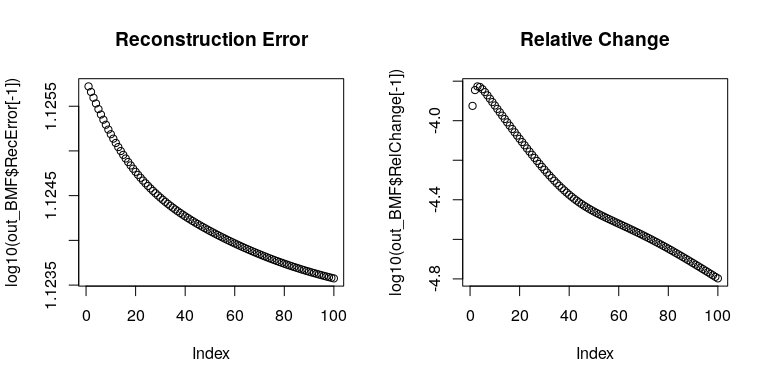The product of $$U$$ and $$V$$ shows whether the original data is well-recovered by dNMF.

recX <- out_BMF$U %*% t(out_BMF$V)
layout(t(1:2))
image.plot(X, main="Original Data", legend.mar=8)
image.plot(recX, main="Reconstructed Data (BMF)", legend.mar=8)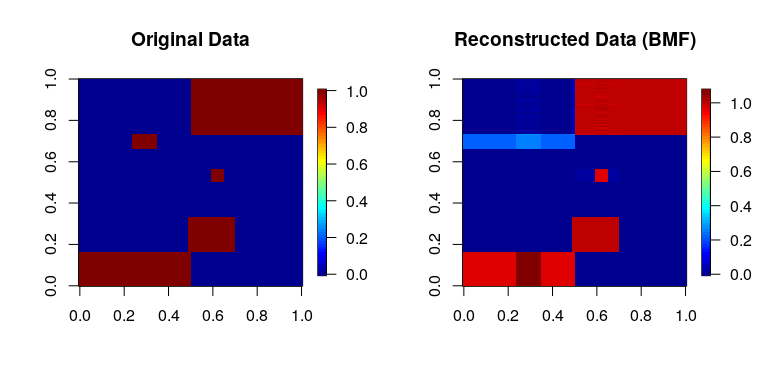The histograms of $$U$$ and $$V$$ show that both $$U$$ and $$V$$ take values close to 0 and 1.

layout(t(1:2))
hist(out_BMF$U, breaks=100) hist(out_BMF$V, breaks=100)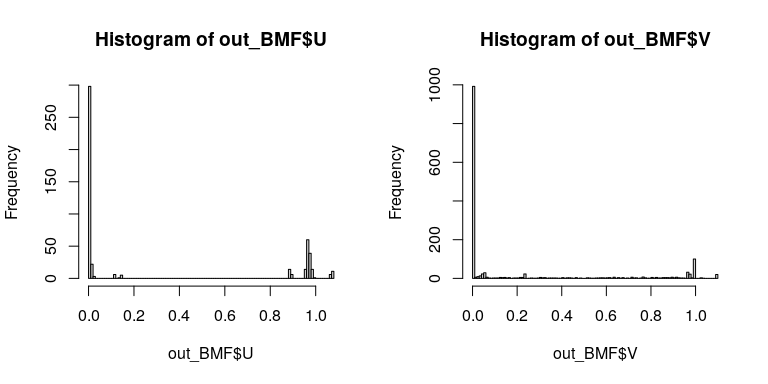Note that these $$U$$ and $$V$$ do not always take the values of 0 and 1 completely. This is because the binarization in BMF is based on the regularization to softly set the values as close to {0,1} as possible, and is not a hard binarization.

head(out_BMF$U) ## [,1] [,2] [,3] [,4] [,5] ## [1,] 0.979044 0 0 3.765852e-104 0 ## [2,] 0.979044 0 0 4.277338e-106 0 ## [3,] 0.979044 0 0 2.301292e-104 0 ## [4,] 0.979044 0 0 1.337948e-104 0 ## [5,] 0.979044 0 0 9.347010e-105 0 ## [6,] 0.979044 0 0 8.698401e-105 0 head(out_BMF$V)
##           [,1]         [,2] [,3]         [,4]          [,5]
## [1,] 0.9988044 1.072698e-56    0 0.0013906118  0.000000e+00
## [2,] 0.9988248 5.888980e-54    0 0.0003300704 1.735858e-209
## [3,] 0.9988038 4.861490e-57    0 0.0014216661  0.000000e+00
## [4,] 0.9988042 5.637380e-58    0 0.0014015649  0.000000e+00
## [5,] 0.9988029 1.288196e-54    0 0.0014663748  0.000000e+00
## [6,] 0.9988025 6.724228e-58    0 0.0014901051  0.000000e+00

If you want to get the {0,1} values, use the round function as below:

head(round(out_BMF$U, 0)) ## [,1] [,2] [,3] [,4] [,5] ## [1,] 1 0 0 0 0 ## [2,] 1 0 0 0 0 ## [3,] 1 0 0 0 0 ## [4,] 1 0 0 0 0 ## [5,] 1 0 0 0 0 ## [6,] 1 0 0 0 0 head(round(out_BMF$V, 0))
##      [,1] [,2] [,3] [,4] [,5]
## [1,]    1    0    0    0    0
## [2,]    1    0    0    0    0
## [3,]    1    0    0    0    0
## [4,]    1    0    0    0    0
## [5,]    1    0    0    0    0
## [6,]    1    0    0    0    0

# Semi-Binary Matrix Factorization (SBMF)

Next, we consider the approximation of a non-negative data matrix $$X$$ ($$N \times M$$) as the matrix product of binary matrix $$U$$ ($$N \times J$$) and non-negative matrix $$V$$ ($$M \times J$$):

$X \approx U V' \ \mathrm{s.t.}\ U \in \{0,1\}, V \geq 0$

Here, we define this formalization as semi-binary matrix factorization (SBMF). SBMF can capture discrete patterns from a non-negative matrix.

To demonstrate SBMF, next we use a non-negative matrix from the nnTensor package.

suppressMessages(library("nnTensor"))
X2 <- nnTensor::toyModel("NMF")

You will see that there are five blocks in the data matrix as follows.

image.plot(X2, main="Original Data", legend.mar=8)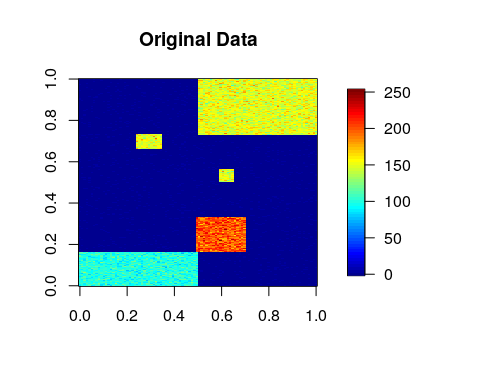## Basic Usage

Switching from BMF to SBMF is quite easy; SBMF is achieved by specifying the binary regularization parameter as a large value like below:

set.seed(123456)
out_SBMF <- dNMF(X2, Bin_U=1E+6, J=5)
str(out_SBMF, 2)
## List of 6
##  $U : num [1:100, 1:5] 0.978 0.984 0.985 0.988 0.977 ... ##$ V            : num [1:300, 1:5] 98.5 100.7 100 101.5 100.6 ...
##  $RecError : Named num [1:101] 1.00e-09 2.99e+03 2.92e+03 2.83e+03 2.77e+03 ... ## ..- attr(*, "names")= chr [1:101] "offset" "1" "2" "3" ... ##$ TrainRecError: Named num [1:101] 1.00e-09 2.99e+03 2.92e+03 2.83e+03 2.77e+03 ...
##   ..- attr(*, "names")= chr [1:101] "offset" "1" "2" "3" ...
##  $TestRecError : Named num [1:101] 1e-09 0e+00 0e+00 0e+00 0e+00 0e+00 0e+00 0e+00 0e+00 0e+00 ... ## ..- attr(*, "names")= chr [1:101] "offset" "1" "2" "3" ... ##$ RelChange    : Named num [1:101] 1.00e-09 2.60e-01 2.44e-02 3.18e-02 2.27e-02 ...
##   ..- attr(*, "names")= chr [1:101] "offset" "1" "2" "3" ...

RecError and RelChange can be used to diagnose whether the calculation is converged or not.

layout(t(1:2))
plot(log10(out_SBMF$RecError[-1]), type="b", main="Reconstruction Error") plot(log10(out_SBMF$RelChange[-1]), type="b", main="Relative Change")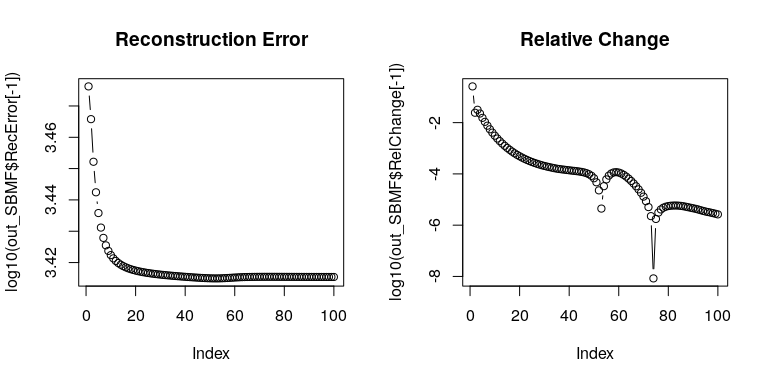The product of $$U$$ and $$V$$ shows whether the original data is well-recovered by dNMF.

recX2 <- out_SBMF$U %*% t(out_SBMF$V)
layout(t(1:2))
image.plot(X2, main="Original Data", legend.mar=8)
image.plot(recX2, main="Reconstructed Data (SBMF)", legend.mar=8)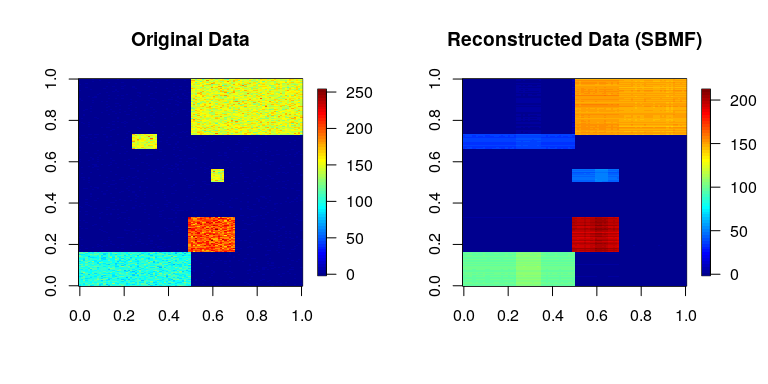The histograms of $$U$$ and $$V$$ show that $$U$$ looks binary but $$V$$ does not.

layout(t(1:2))
hist(out_SBMF$U, breaks=100) hist(out_SBMF$V, breaks=100)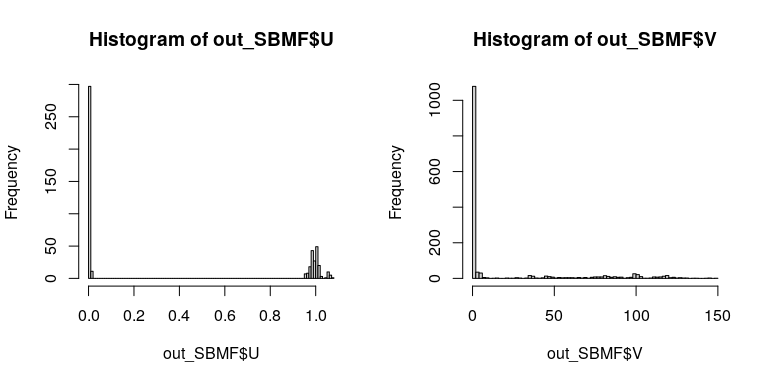# Semi-Ternary Matrix Factorization (STMF)

Finally, we expand the binary regularization to ternary regularization to take {0,1,2} values as below:

$X \approx U V' \ \mathrm{s.t.}\ U \in \{0,1,2\}, V \geq 0,$ where $$X$$ ($$N \times M$$) is a non-negative data matrix, $$U$$ ($$N \times J$$) is a ternary matrix, and $$V$$ ($$M \times J$$) is a non-negative matrix.

## Basic Usage

STMF is achieved by specifying the ternary regularization parameter as a large value like the below:

set.seed(123456)
out_STMF <- dNMF(X2, Ter_U=1E+6, J=5)
str(out_STMF, 2)
## List of 6
##  $U : num [1:100, 1:5] 2.02 2.02 2.02 2.02 2.02 ... ##$ V            : num [1:300, 1:5] 48.7 49.8 49.5 50.2 49.8 ...
##  $RecError : Named num [1:101] 1.00e-09 2.52e+03 2.58e+03 2.59e+03 2.58e+03 ... ## ..- attr(*, "names")= chr [1:101] "offset" "1" "2" "3" ... ##$ TrainRecError: Named num [1:101] 1.00e-09 2.52e+03 2.58e+03 2.59e+03 2.58e+03 ...
##   ..- attr(*, "names")= chr [1:101] "offset" "1" "2" "3" ...
##  $TestRecError : Named num [1:101] 1e-09 0e+00 0e+00 0e+00 0e+00 0e+00 0e+00 0e+00 0e+00 0e+00 ... ## ..- attr(*, "names")= chr [1:101] "offset" "1" "2" "3" ... ##$ RelChange    : Named num [1:101] 1.00e-09 1.22e-01 2.12e-02 3.09e-03 2.03e-03 ...
##   ..- attr(*, "names")= chr [1:101] "offset" "1" "2" "3" ...

RecError and RelChange can be used to diagnose whether the calculation is converging or not.

layout(t(1:2))
plot(log10(out_STMF$RecError[-1]), type="b", main="Reconstruction Error") plot(log10(out_STMF$RelChange[-1]), type="b", main="Relative Change")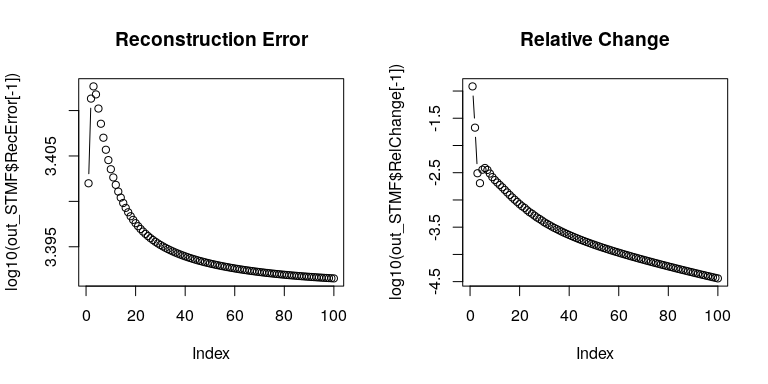The product of $$U$$ and $$V$$ shows that the original data is well-recovered by dNMF.

recX <- out_STMF$U %*% t(out_STMF$V)
layout(t(1:2))
image.plot(X2, main="Original Data", legend.mar=8)
image.plot(recX, main="Reconstructed Data (STMF)", legend.mar=8)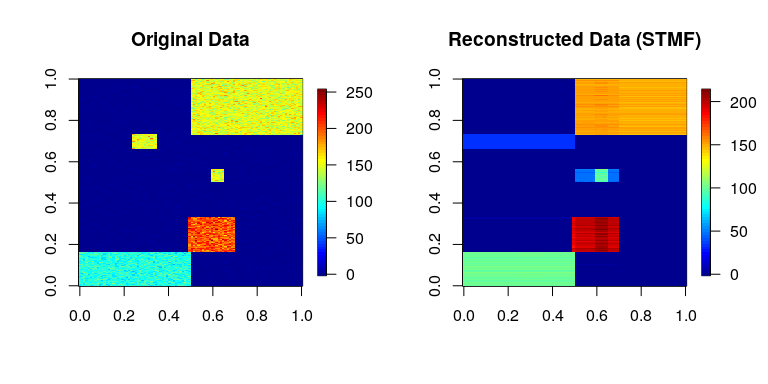The histograms of $$U$$ and $$V$$ show that $$U$$ looks ternary but $$V$$ does not.

layout(t(1:2))
hist(out_STMF$U, breaks=100) hist(out_STMF$V, breaks=100)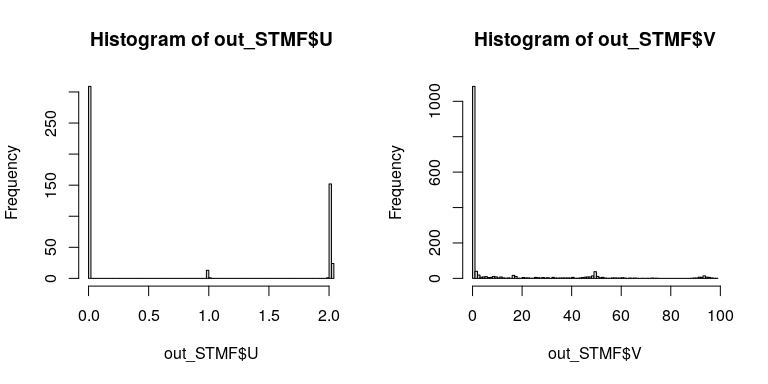# Session Information

## R version 4.3.0 (2023-04-21)
## Platform: x86_64-pc-linux-gnu (64-bit)
## Running under: Ubuntu 22.04.2 LTS
##
## Matrix products: default
## LAPACK: /usr/lib/x86_64-linux-gnu/openblas-pthread/libopenblasp-r0.3.20.so;  LAPACK version 3.10.0
##
## locale:
##   LC_CTYPE=en_US.UTF-8       LC_NUMERIC=C
##   LC_TIME=en_US.UTF-8        LC_COLLATE=C
##   LC_MONETARY=en_US.UTF-8    LC_MESSAGES=en_US.UTF-8
##   LC_PAPER=en_US.UTF-8       LC_NAME=C
##  LC_MEASUREMENT=en_US.UTF-8 LC_IDENTIFICATION=C
##
## time zone: Etc/UTC
## tzcode source: system (glibc)
##
## attached base packages:
##  stats     graphics  grDevices utils     datasets  methods   base
##
## other attached packages:
##  nnTensor_1.1.13   fields_14.1       viridis_0.6.3     viridisLite_0.4.2
##  spam_2.9-1        dcTensor_1.2.0
##
## loaded via a namespace (and not attached):
##   gtable_0.3.3       jsonlite_1.8.5     highr_0.10         dplyr_1.1.2
##   compiler_4.3.0     maps_3.4.1         Rcpp_1.0.10        tagcloud_0.6
##   plot3D_1.4         tidyselect_1.2.0   gridExtra_2.3      jquerylib_0.1.4
##  scales_1.2.1       yaml_2.3.7         fastmap_1.1.1      ggplot2_3.4.2
##  R6_2.5.1           tcltk_4.3.0        generics_0.1.3     knitr_1.43
##  MASS_7.3-60        misc3d_0.9-1       dotCall64_1.0-2    tibble_3.2.1
##  munsell_0.5.0      RColorBrewer_1.1-3 bslib_0.5.0        pillar_1.9.0
##  rlang_1.1.1        utf8_1.2.3         cachem_1.0.8       xfun_0.39
##  sass_0.4.6         cli_3.6.1          magrittr_2.0.3     digest_0.6.31
##  grid_4.3.0         rTensor_1.4.8      lifecycle_1.0.3    vctrs_0.6.2
##  evaluate_0.21      glue_1.6.2         fansi_1.0.4        colorspace_2.1-0
##  rmarkdown_2.22     tools_4.3.0        pkgconfig_2.0.3    htmltools_0.5.5